Disclosure: This post may contain affiliate links, meaning when you click the links and make a purchase, we receive a commission.

# The Different Ways of Counting in Excel

There is a total of five functions used for counting, predominantly in Excel. These are namely the COUNT Function, the COUNTA Function, the COUNTIF Function, the COUNTIFS Function and the COUNTBLANK Function.

The COUNT Function is used to determine the number of cells that contain numbers or a number of numbers in a list. It is the simplest of the counting functions. Dates are also counted when using this function. The syntax for the COUNT Function is: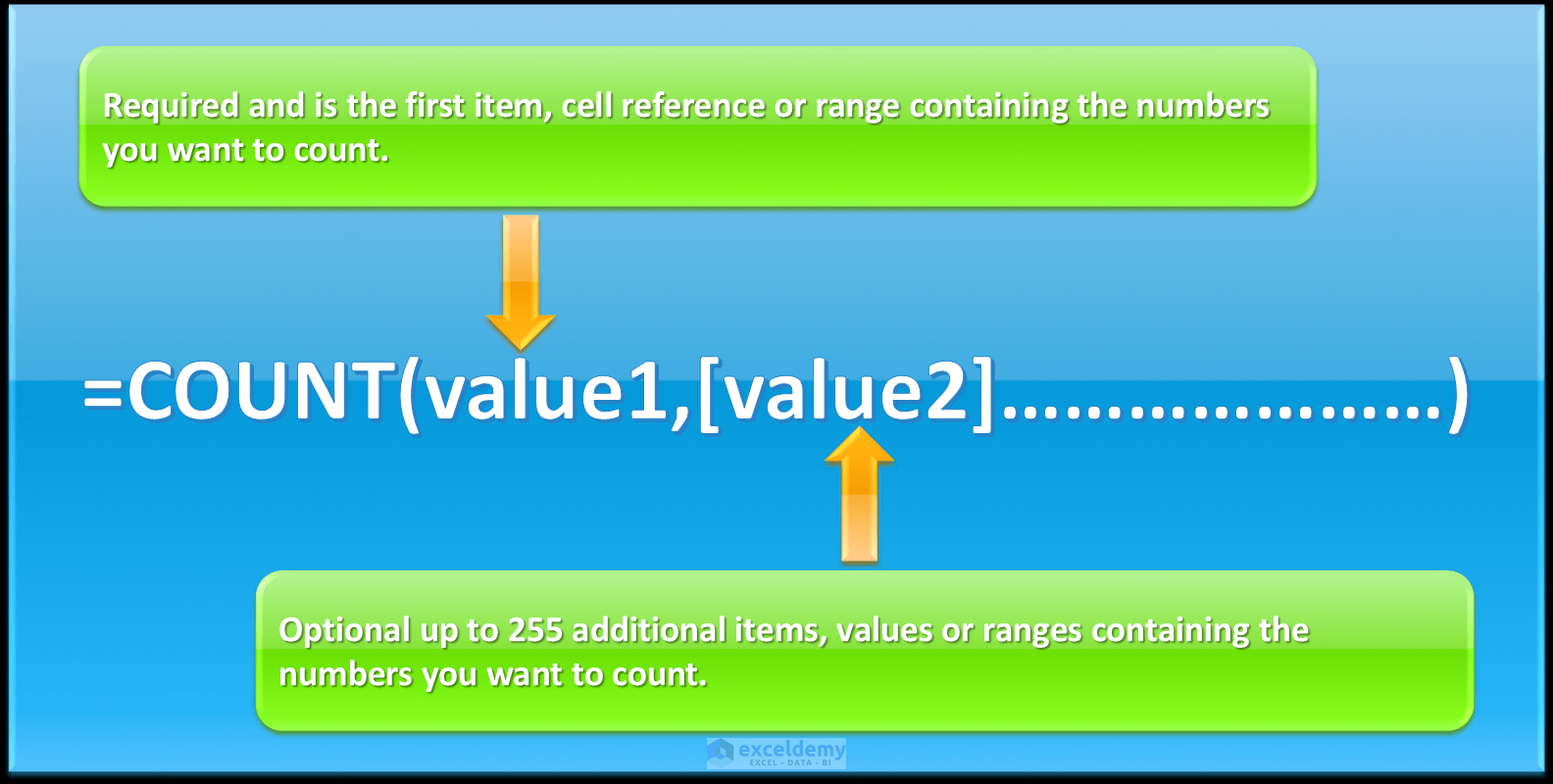The COUNTA Function is used to count the number of cells, within a particular range that is not empty. This function counts numbers, error values, logical formulas and formulas that return an empty text string. The syntax for the COUNTA Function is: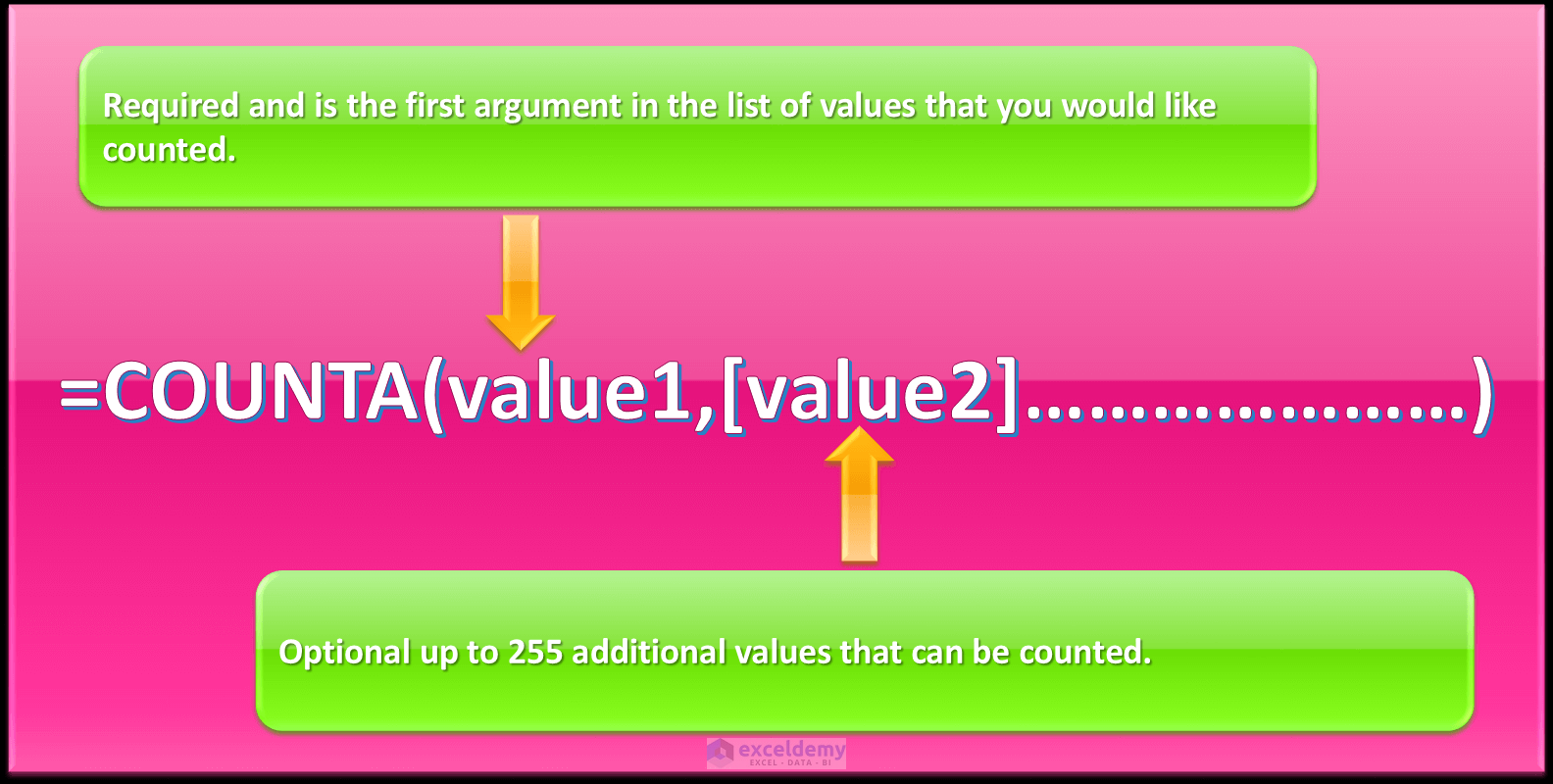The COUNTIF Function beings conditional logic into the equation and counts the number of cells that meet specific criteria. The syntax for the COUNTIF Function is: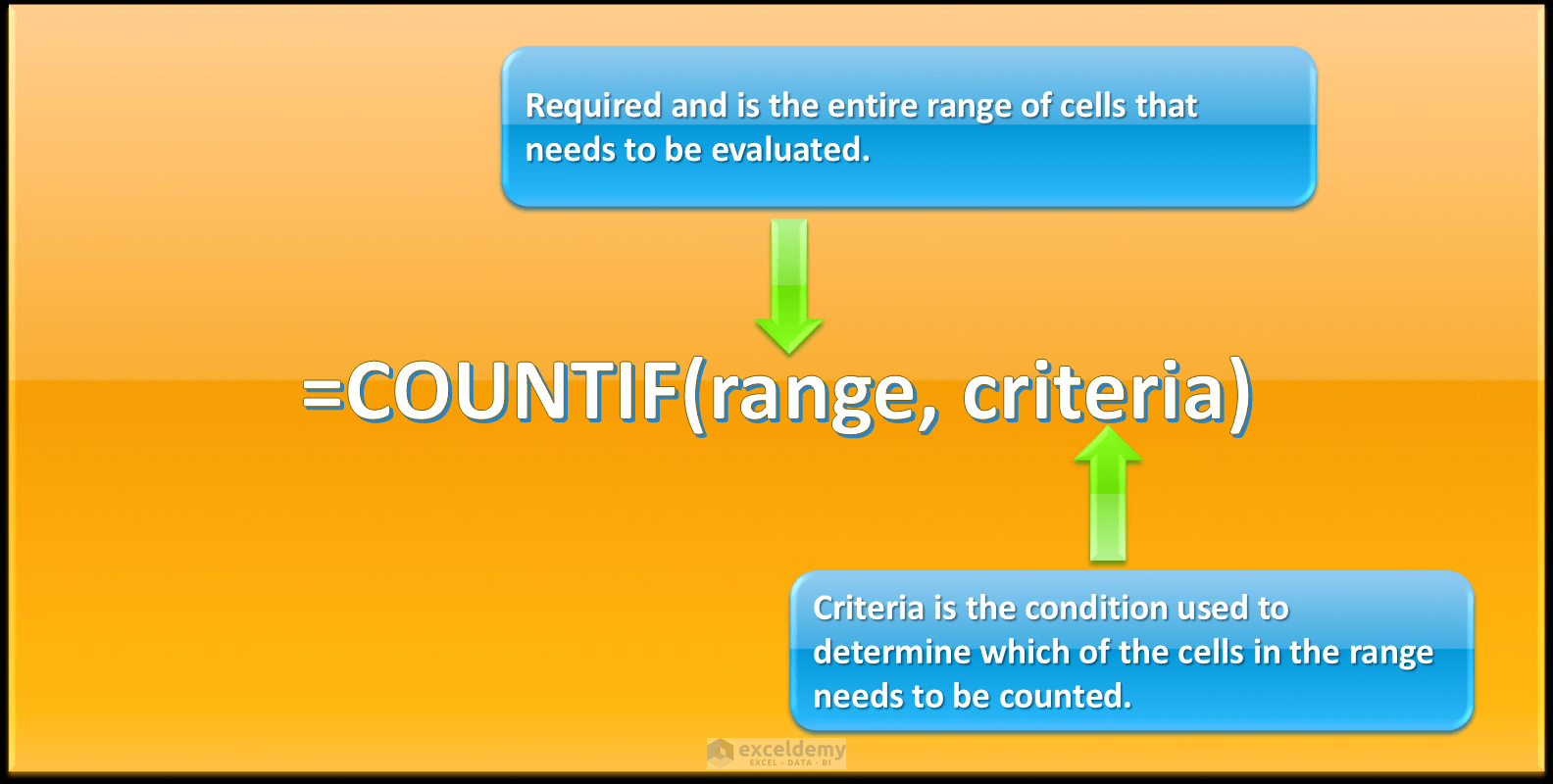The COUNTIFS Function is similar to the COUNTIF Function, except it’s used to count the number of cells in a range that meet multiple criteria. All the criteria have to be met for COUNTIFS to count that specific value. So, if one thinks of this using logical formula logic all conditions set have to evaluate to true for a row to be counted.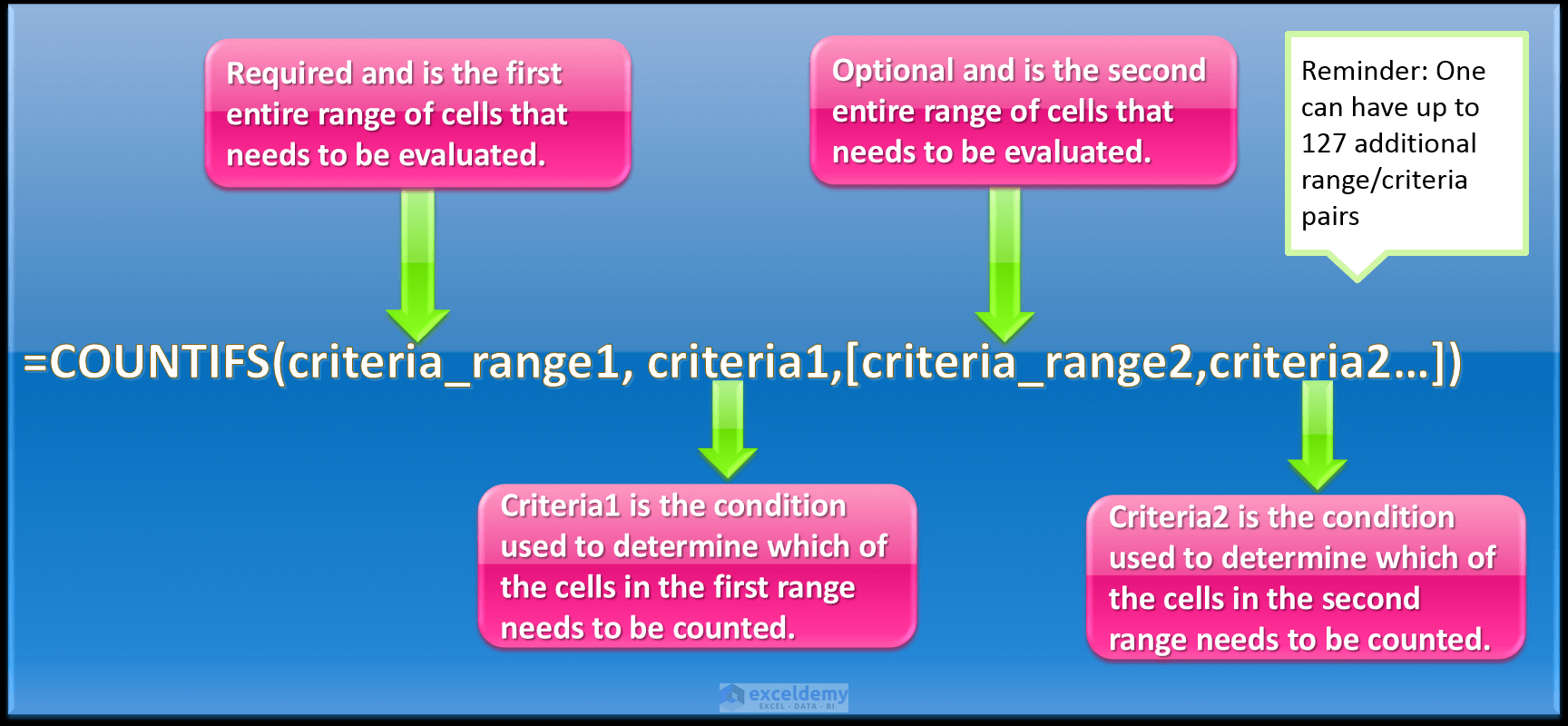The COUNTBLANK Function counts the number of blank cells in a specified range. The syntax for the COUNTBLANK Function is: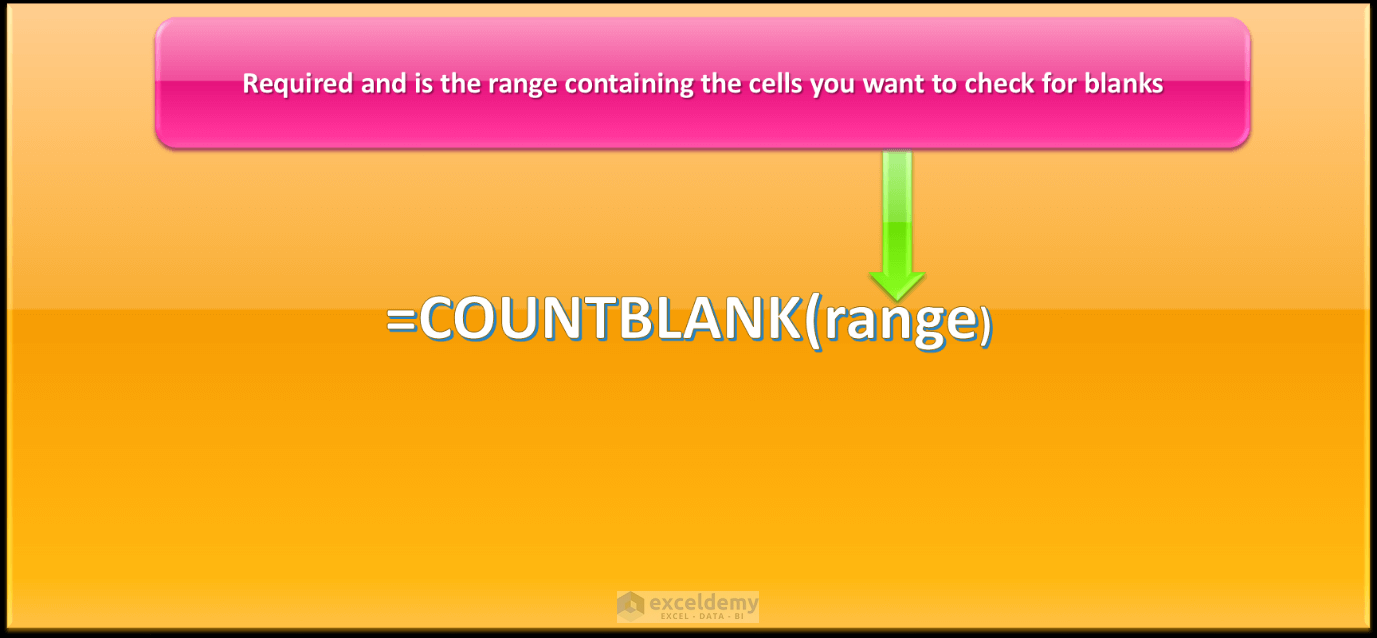So let’s get started with a few simple examples illustrating the use of the different counting functions in Excel.

Read More: Excel Dynamic Named Range [4 Ways]

## The COUNT Function

There is a mixed list of data that has numbers, text, characters and logical values. We want to determine only the numbers in the list using the COUNT Function.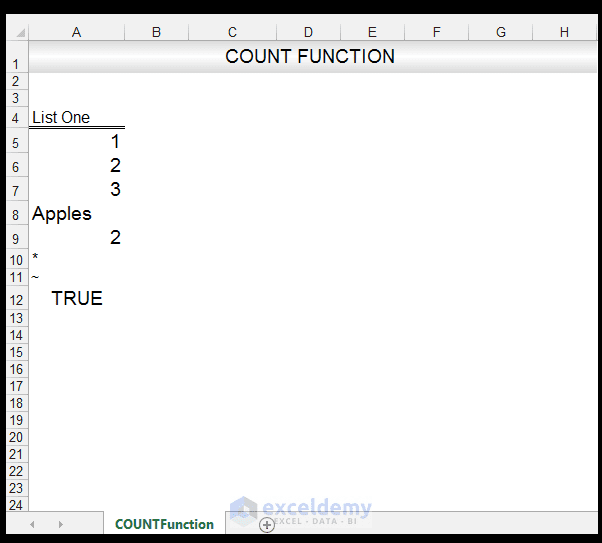1) In order to count the numbers in the cell range A5: A12, we input the following formula in Cell A13:
=COUNT (A5: A12)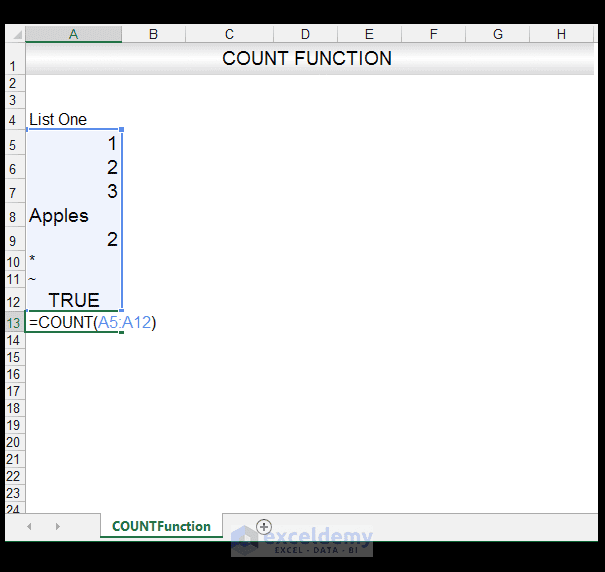2) Upon pressing CTRL-ENTER, we get a value of 4 returned.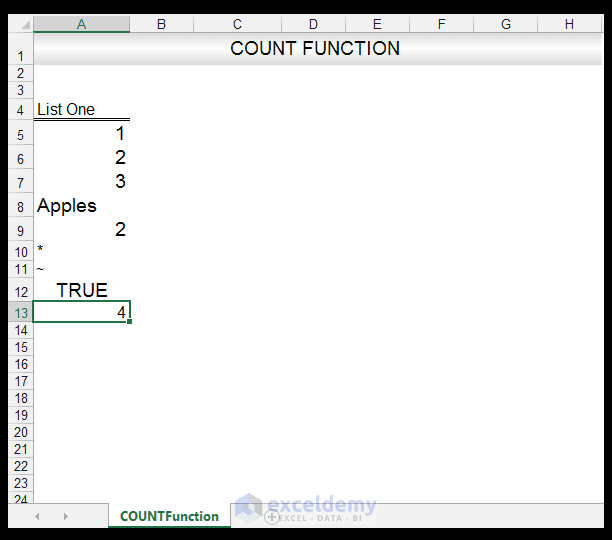### Crossover Tip: One can also use the COUNT Function in MS Word

1) We have a table in Word, with the values in numbers listed underneath the different fruits and we want to count the number of times a number value is listed.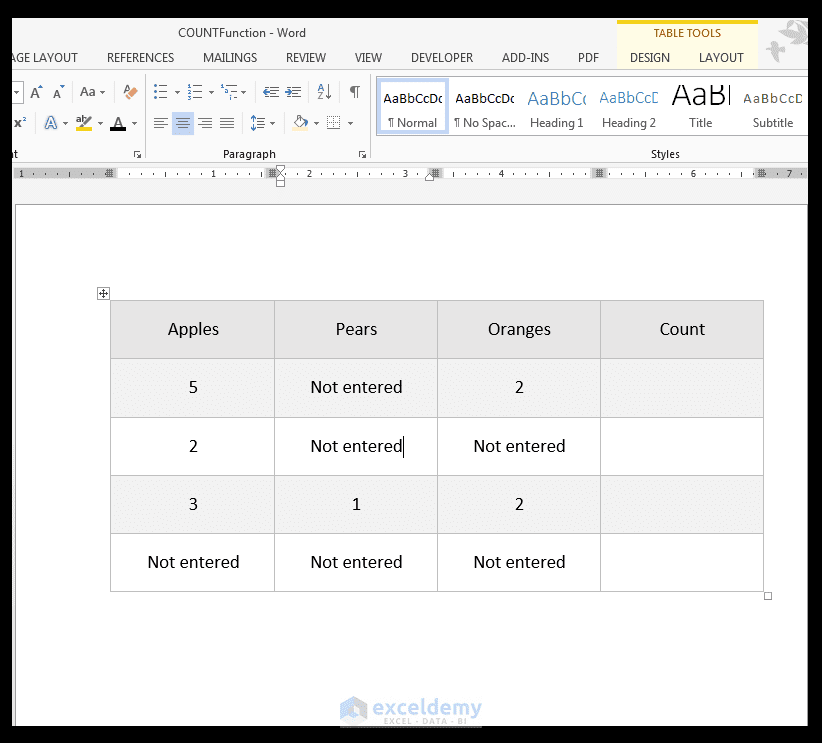2) The way the cell references in a Table work in Word is shown below: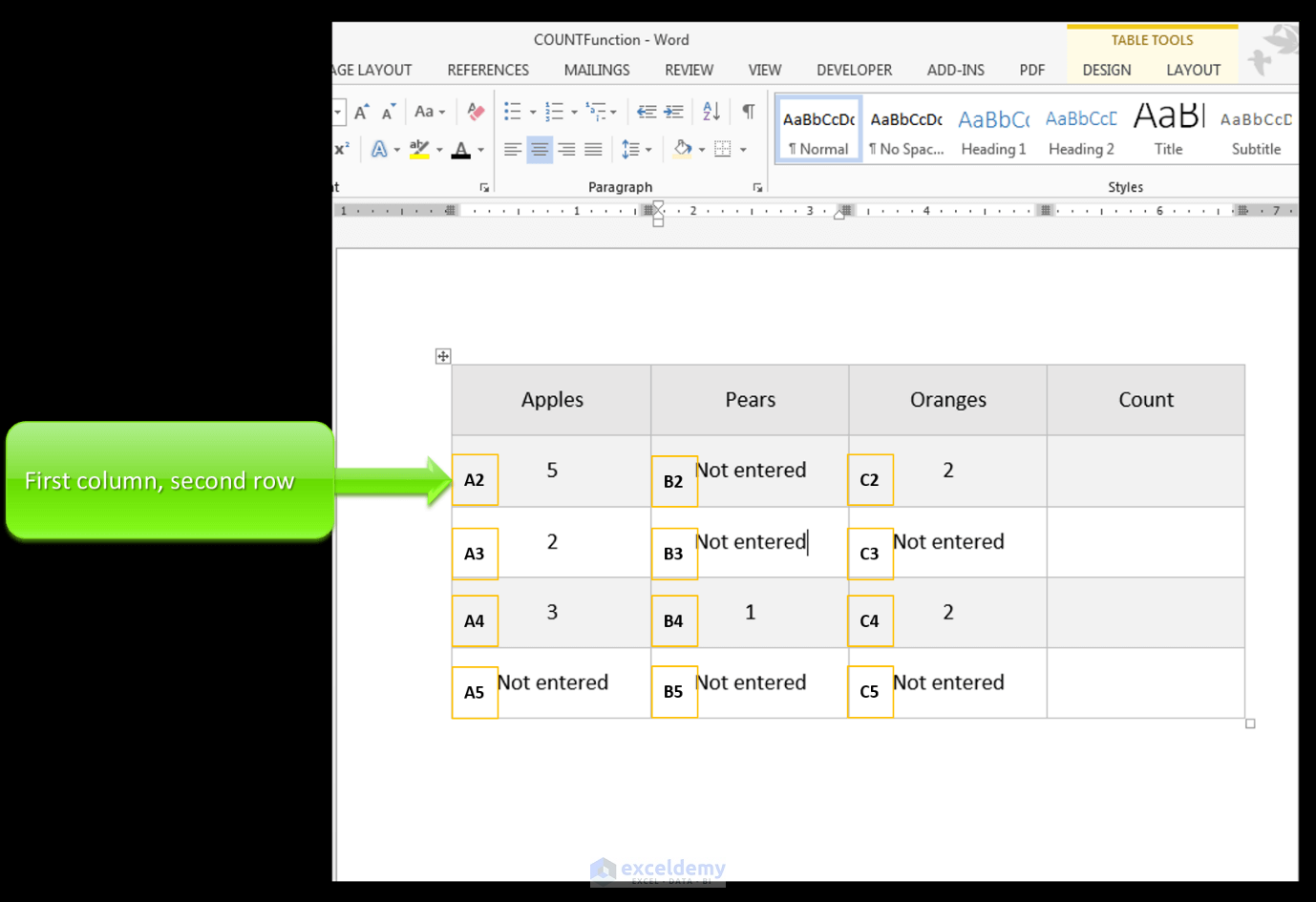3) In order to insert a formula in the last cell D2, with the Table Tools context sensitive menu activated, go to Table Tools>Layout>Data>Formula.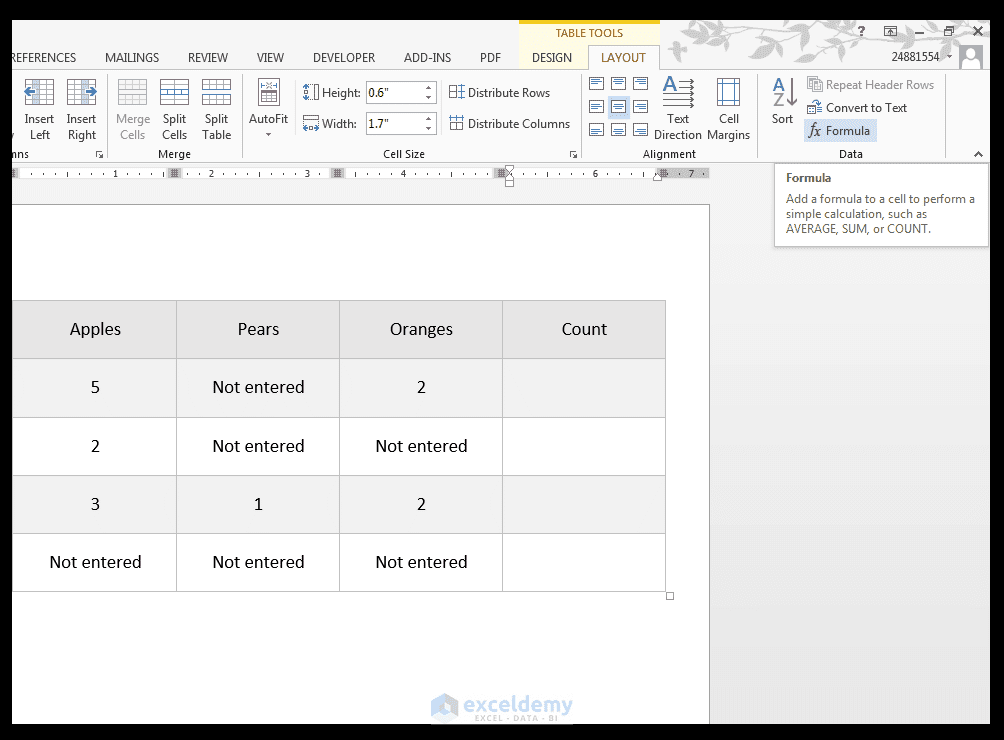4) Enter the formula

=COUNT (A2: C2)

Select a number format and click Ok as shown.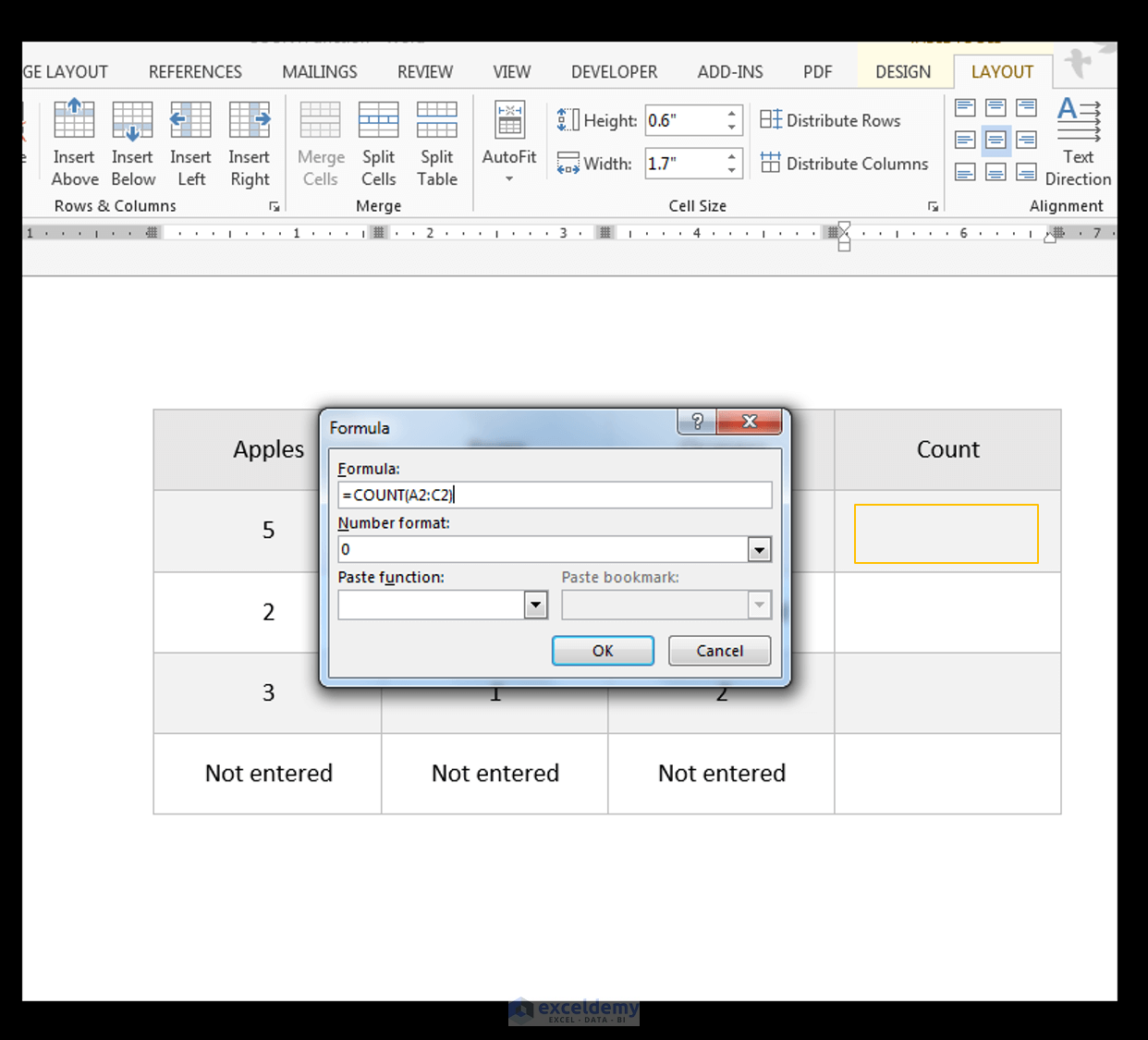5) A value of 2 is delivered from the COUNT Function.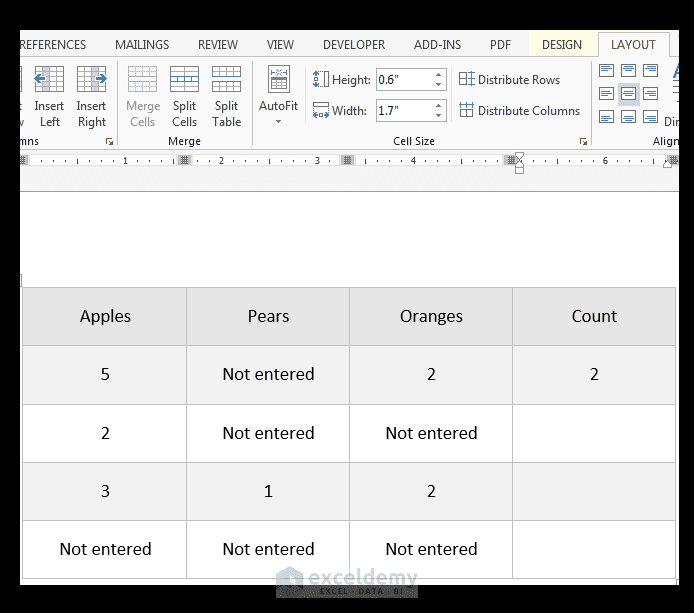6) Doing the same for the rest of the column results in the following as shown below.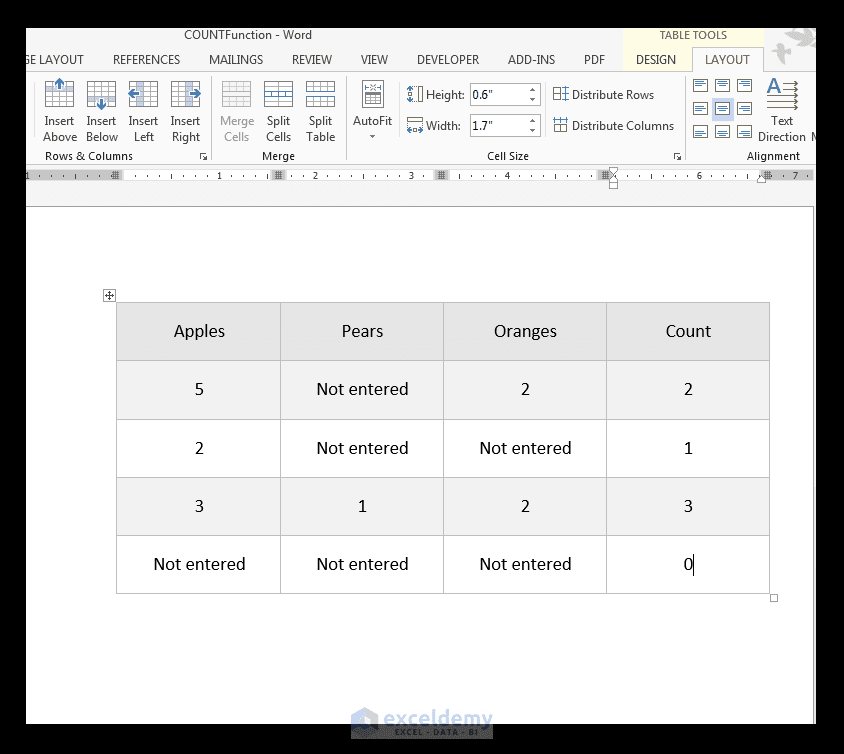7) It is often necessary to manually update formulas in Word, since the formula is calculated in Word at the time of insertion and when the user opens the document containing the formula. So, in order to do this Press CTRL-A on your keyboard and the F9 key and then all the formulas in the Word document will be updated with the current most relevant results.

## The COUNTA Function

There is a mixed list of data that has numbers, text, characters and logical values. We want to determine the cells that are not blank in the list using the COUNTA Function.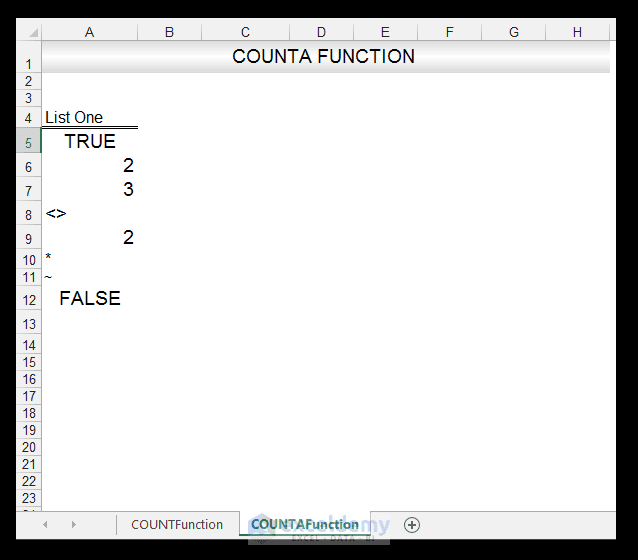1) In order to count the number of cells that are not blank, in the cell range A5: A12, we input the following formula in Cell A13:

=COUNTA (A5: A12)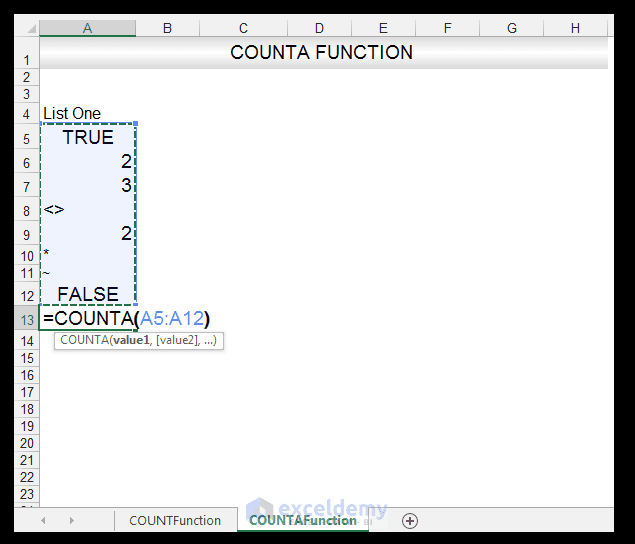2) Upon pressing CTRL-ENTER, we get a value of 8 returned.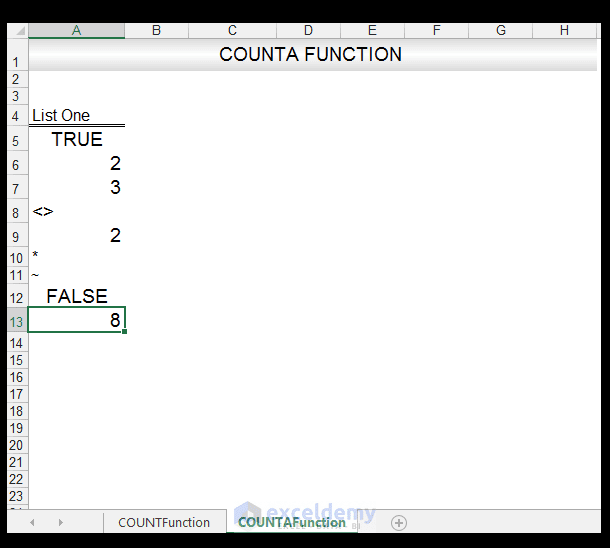Read More: COUNTIF formula in Excel

### Creating Dynamic Ranges with COUNTA

1) In order to create dynamic ranges, one often has to use the COUNTA Function.

2) We have a range in cell A5: A9 called Apples_Sold, which was previously created and named.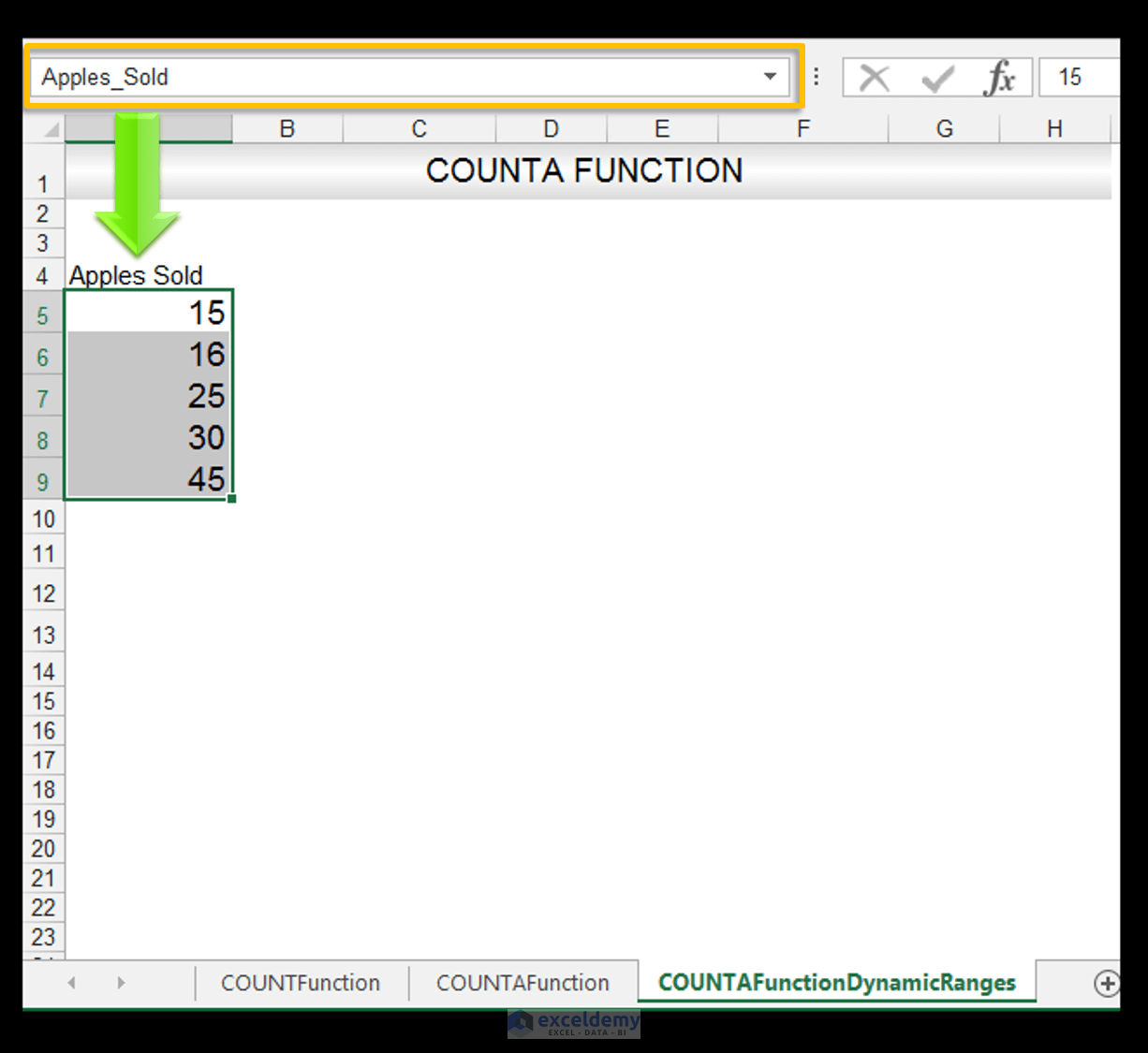3) Now let’s say we wanted to add an extra number in A10, that denotes more Apples sold or data not collected for that particular period, we would, of course, want the range to update automatically, and in order to do this, we can use the COUNTA Function in order to create a dynamic range.

4) Go to Formulas>Defined Names>Name Manager as shown below: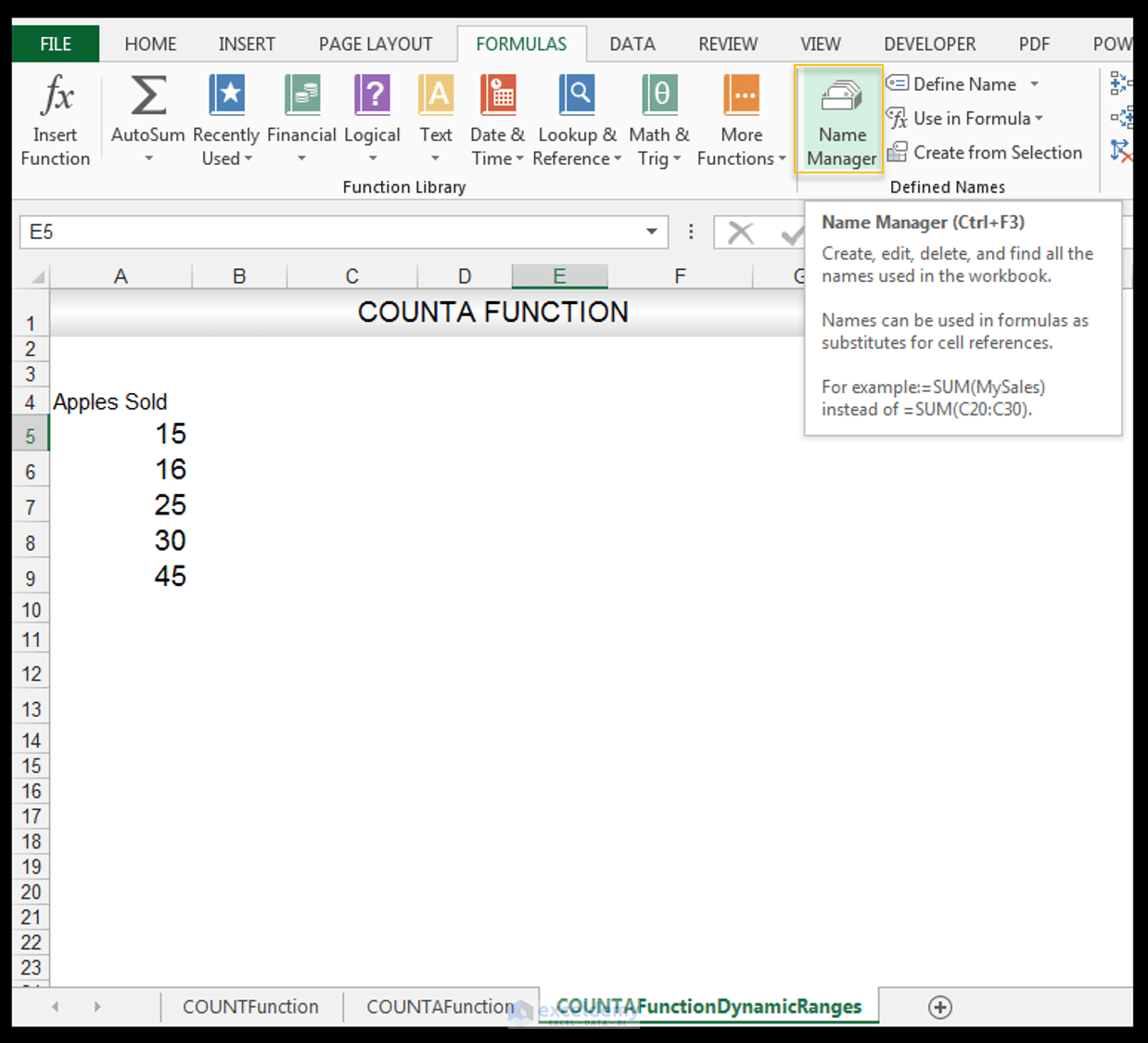5) Make sure the Apples_Sold range is selected and choose Edit as shown.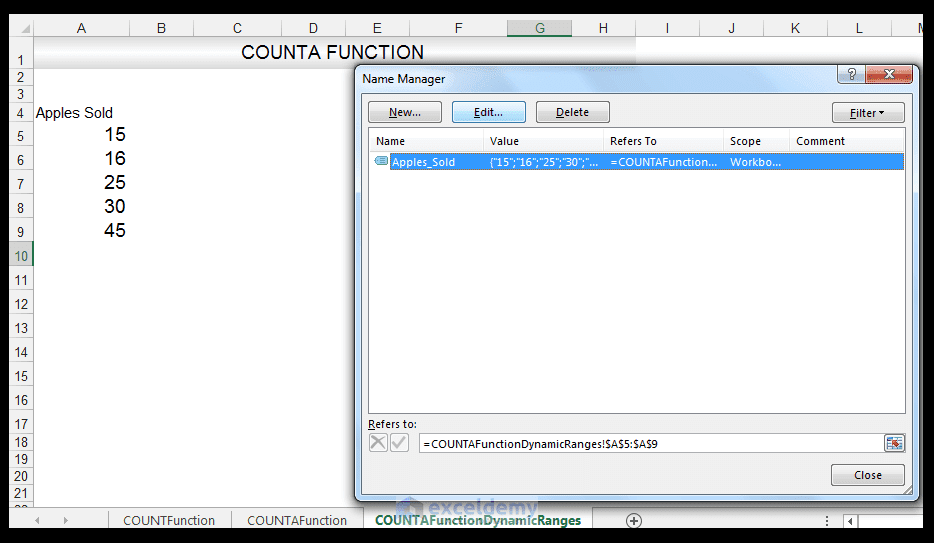6) In the formula bar, type in the following formula:

=OFFSET(\$A\$5,0,0,COUNTA(\$A\$5:\$A\$1048576),1)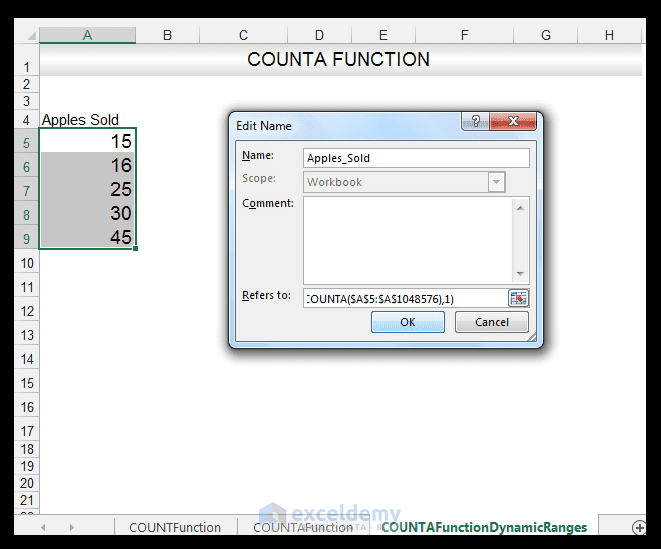7) Press Ok and then Close.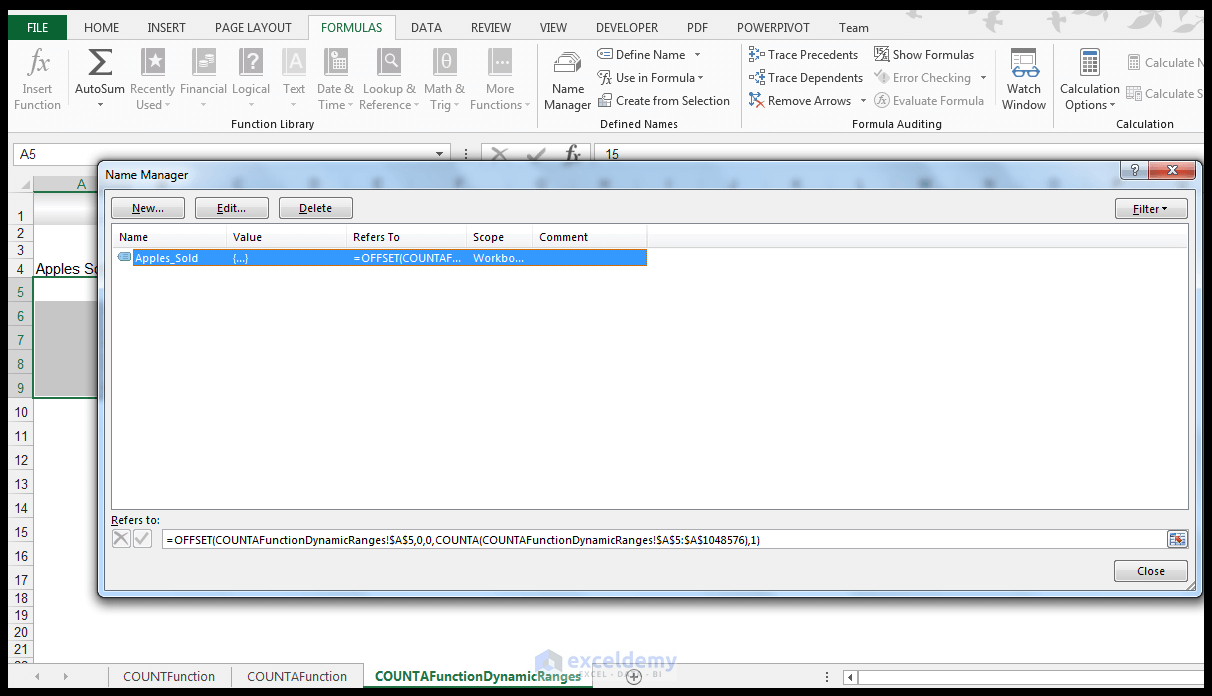8) In Cell E5, input the following formula:

=COUNTA (Apples_Sold)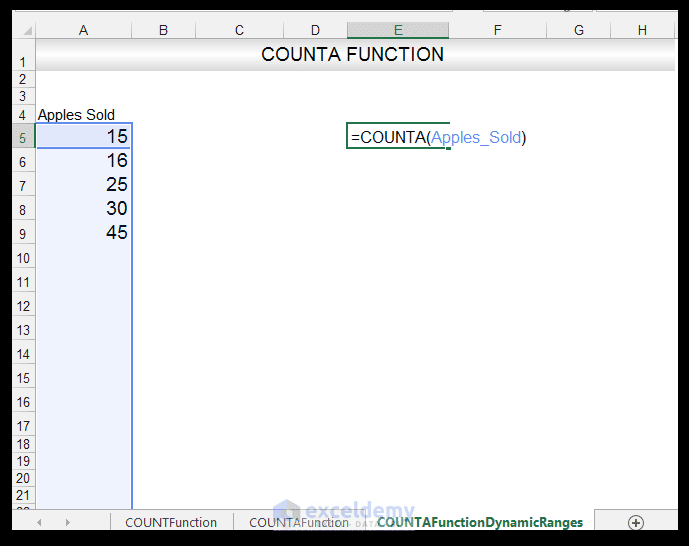9) Upon pressing CTRL-ENTER, the value of 5 is delivered.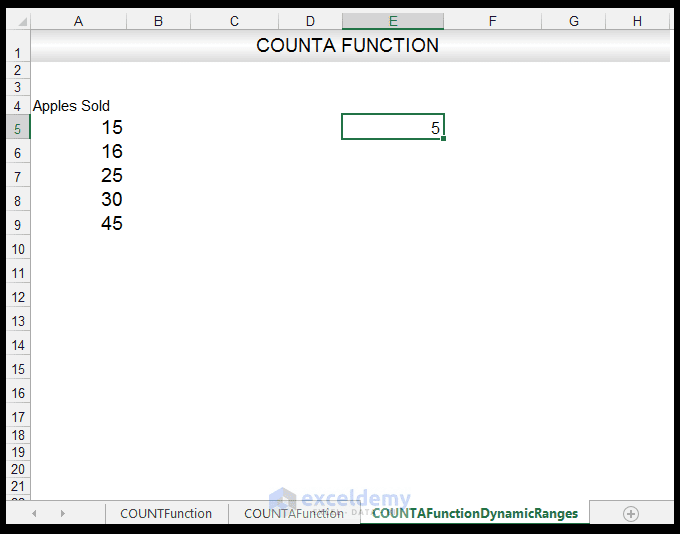10) If you type the value, Not collected in Cell A10, the formula in E5 automatically updates with the new entry since one used the COUNTA Function if you want only numbers to use COUNT instead.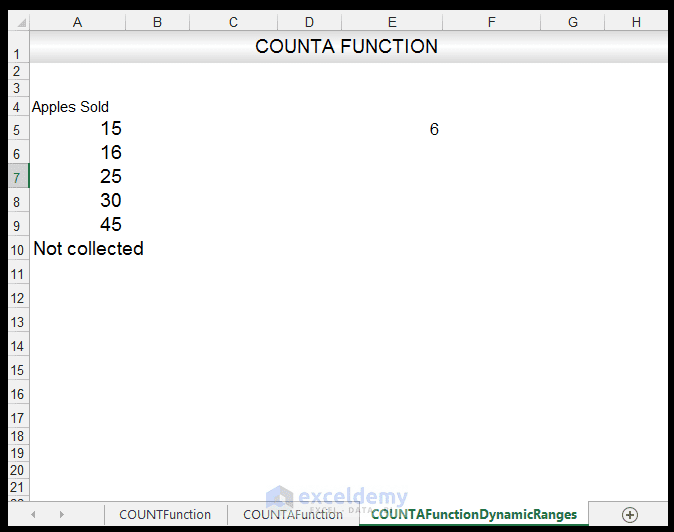Read More: Count Cells that Contain Specific Text in Excel

## The COUNTIF Function

There is a mixed list of data that has different fruits and the corresponding sales of the fruits. We want to count only the values that exceeded \$ 500, this is clearly a condition or criteria attached so we will have to use the COUNTIF Function.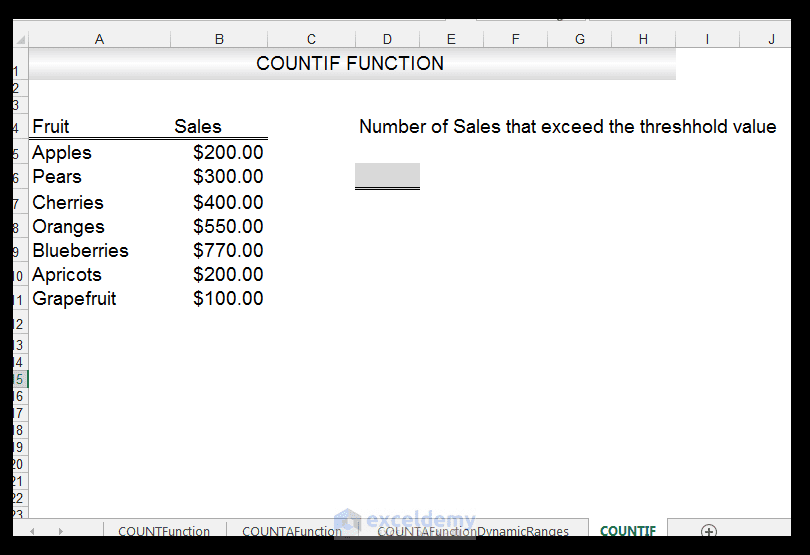1) In order to count the number of cells that have a value greater than \$ 500, in the range cell B5: B11, we input the following formula in Cell D6:

=COUNTIF (B5: B11, “>500”)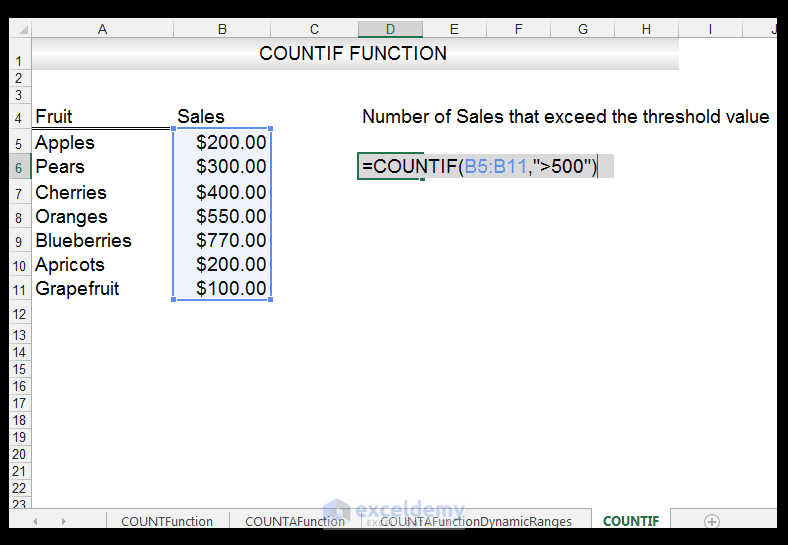2) Upon pressing CTRL-ENTER, we get a value of 2 is returned, so two values, in other words, exceeded the \$ 500 threshold.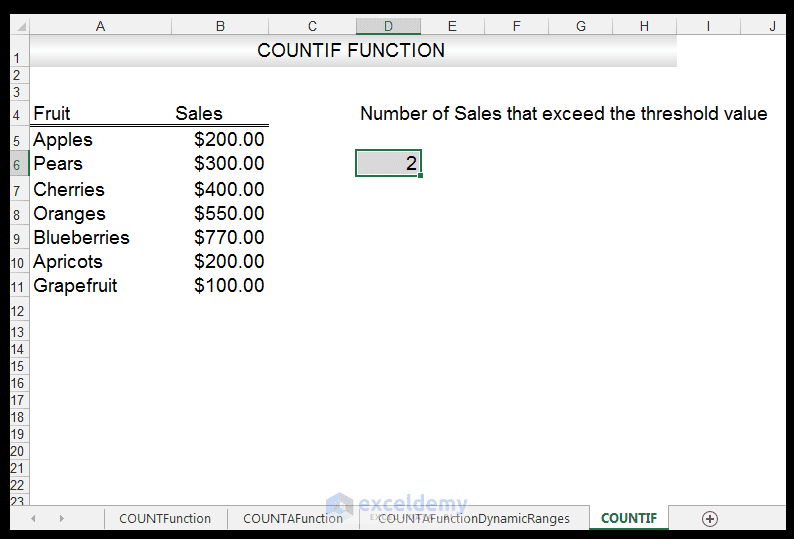## The COUNTIFS Function

There is a mixed list of data that has different fruits and the amounts sold. We want to count only the values of apples, whose sales exceeded \$ 200, so we can use the COUNTIFS Function to accomplish this task since we now have more than one criteria that have to be fulfilled.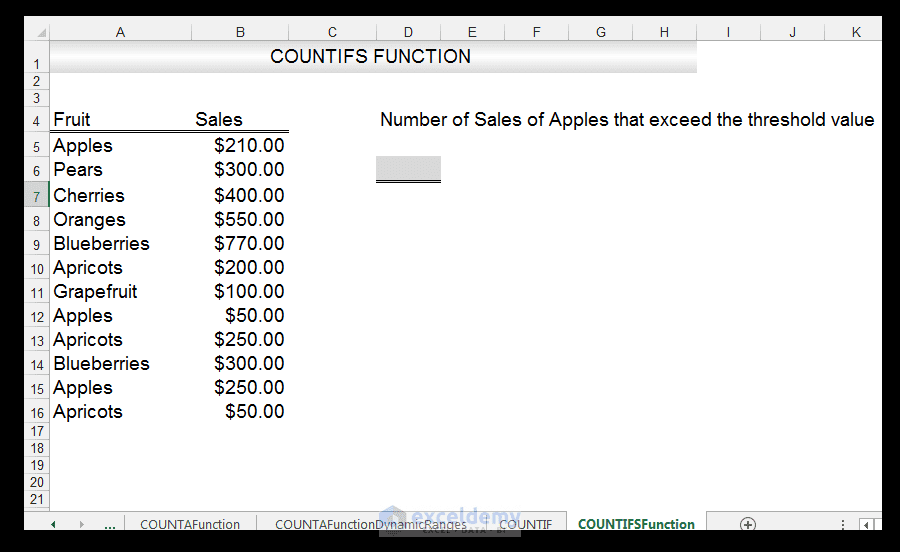1) In order to count the number of cells that have a value of Apples with sales exceeding \$ 200, we input the following formula in Cell D6:

=COUNTIFS(A5:A16,”Apples”,B5:B16,”>200″)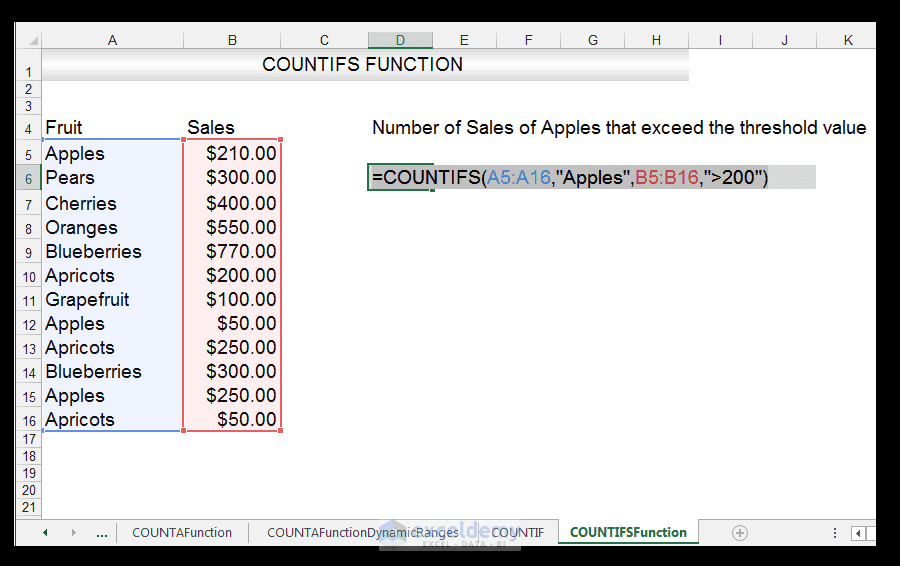2) Upon pressing CTRL-ENTER, we get a value of 2 is returned, so two values, in other words only two values fulfill the requirement of being apples with sales of greater than \$ 200.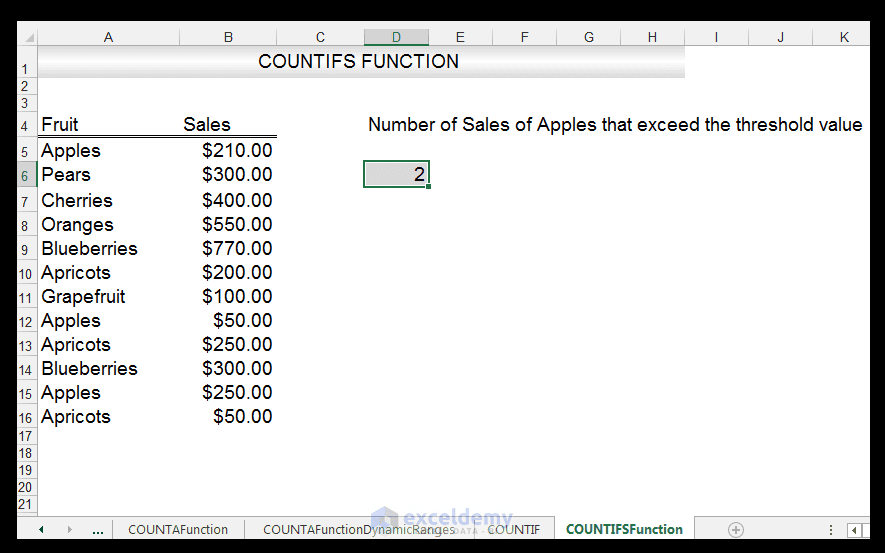### Using Wildcards

1) One can also use Wildcards when summing, counting or using TEXT Functions in order to extend functionality in Excel.

2) In the example used above, we now want count all the fruits starting with the letter A, and whose sales exceed \$ 200.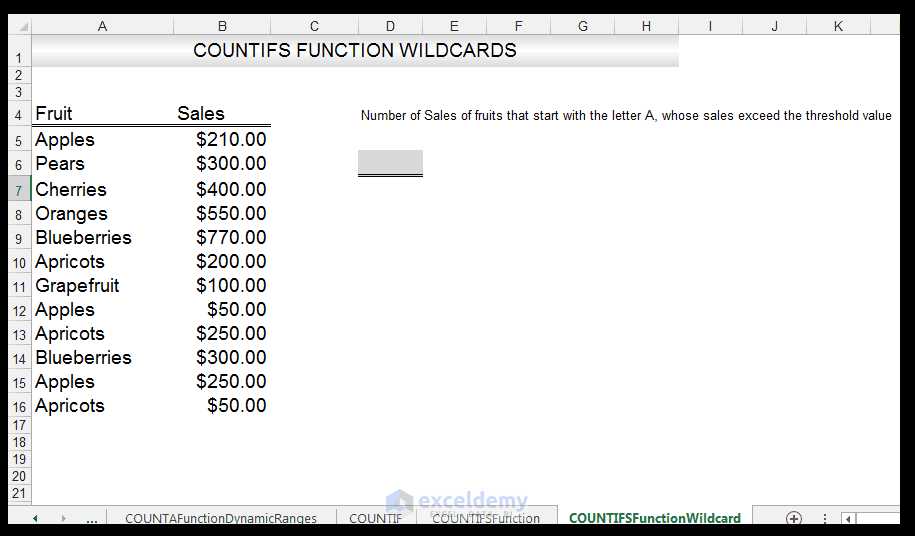3) So in Cell D6, we enter the following formula:

=COUNTIFS(A5:A16,”A*”,B5:B16,”>200″)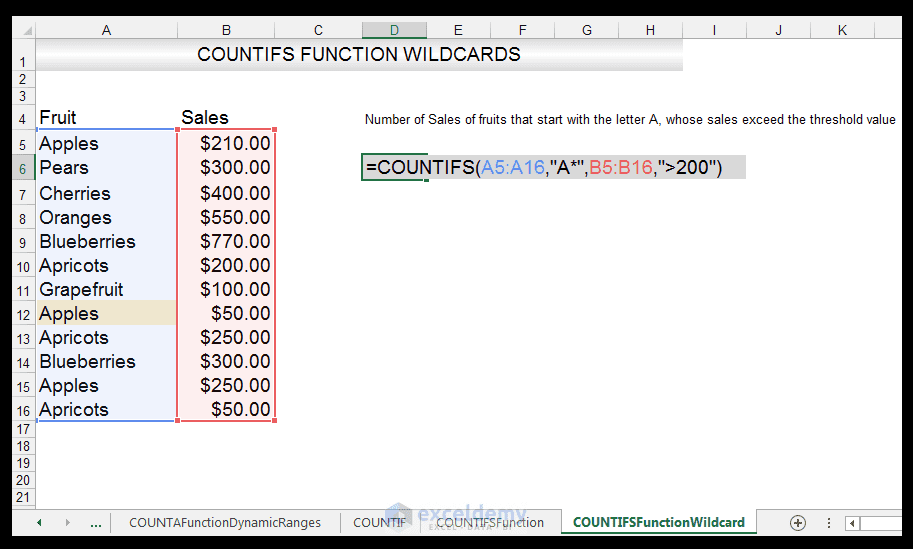4) Upon pressing CTRL-ENTER, we get a value of 3 is returned, so three values, in other words, only three values fulfill the requirement of starting with the letter A, with sales of greater than \$ 200.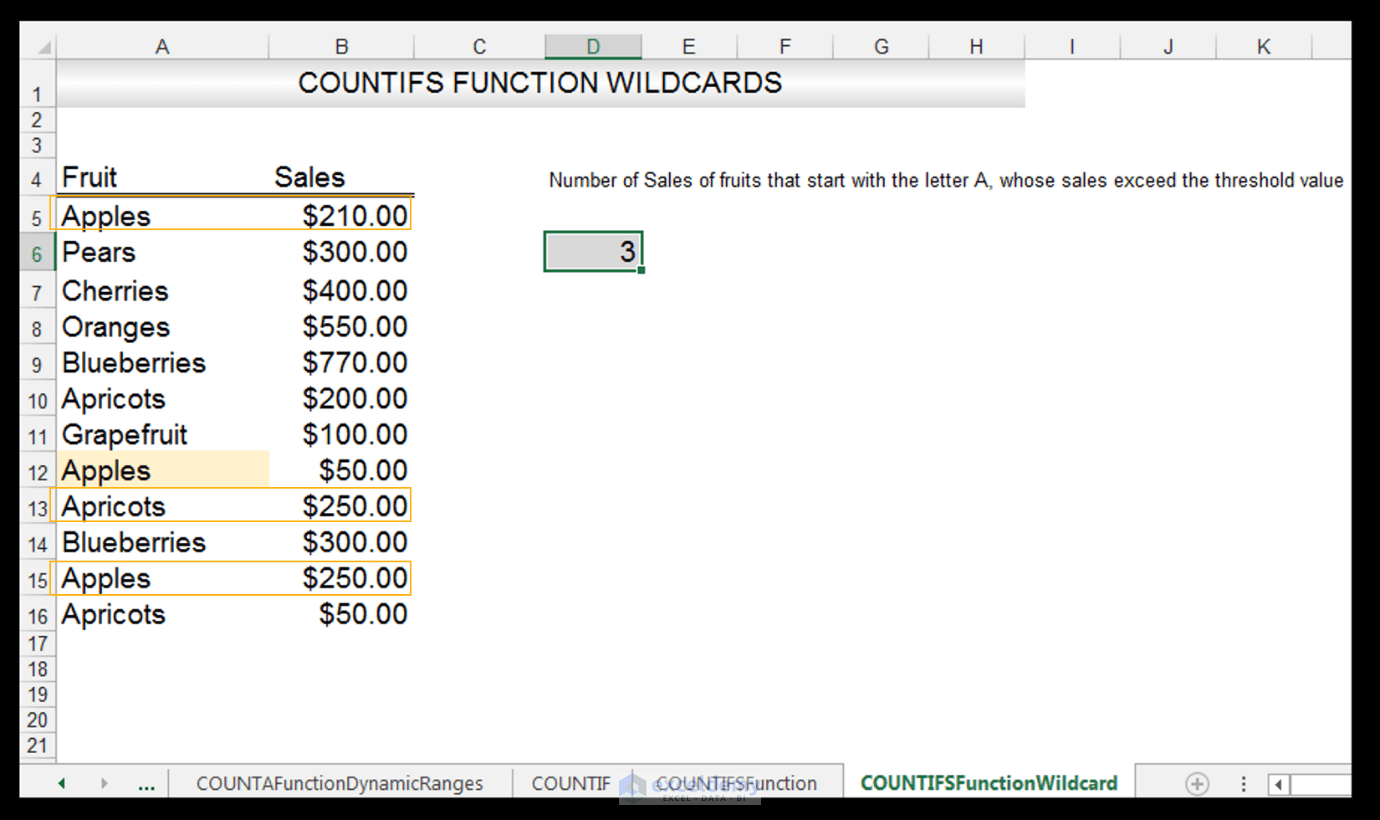When using COUNTIF or COUNTIFS to count certain text values, you may need to first use the CLEAN Function or the TRIM Function to clean the data, since spaces will affect the results. Neither the COUNTIF Function or the COUNTIFS Function are case sensitive so apples should be counted as well as Apples.

Read More: Finding out the number of duplicate rows using COUNTIF formula

## The COUNTBLANK Function

There is a mixed list of data that has different values and a few blank cells, in between and we want to use the COUNTBLANK Function in order to determine the number of blank cells in the range.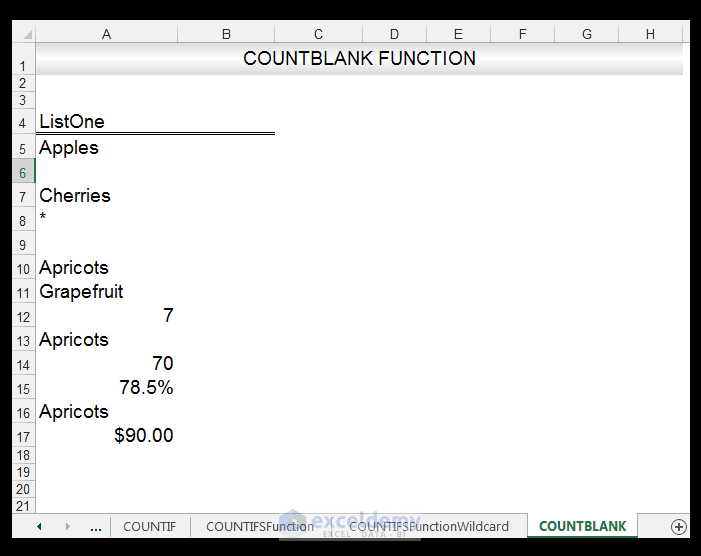1) In order to count the number of blank cells that fall within the range, we first have to turn off Enable iterative calculation. We do this by going to File>Options>Formulas and making sure that the Enable iterative calculations checkbox is clear.

2) So in Cell A18, we enter the following formula:

=COUNTBLANK (A5: A17)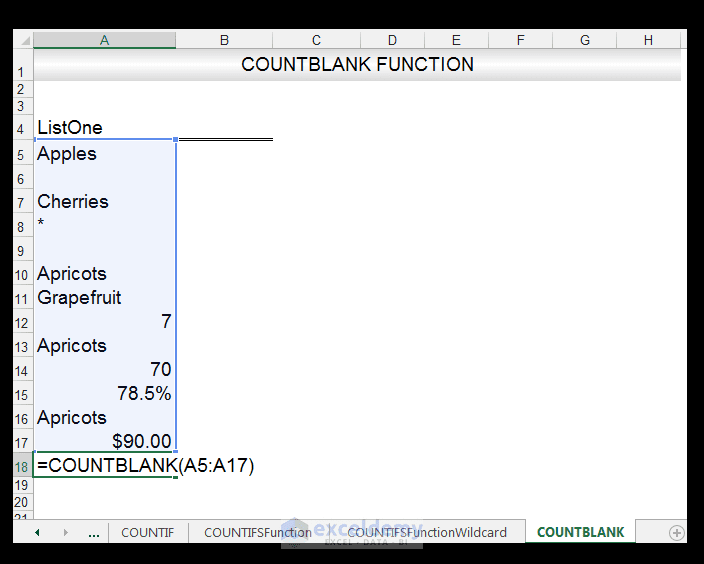3) Upon pressing CTRL-ENTER, we get a value of 2 returned, so of the cells in the range, there were only two blank cells.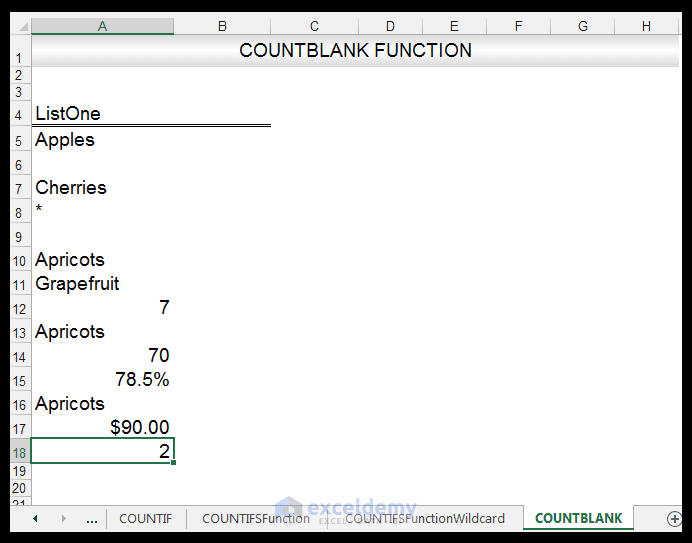### Using Conditional Formatting Example

1) You can also use conditional formatting in order to highlight the blank cells.

2) In order to do this select the range, you want to conditionally format and go to Home>Styles>Conditional Formatting.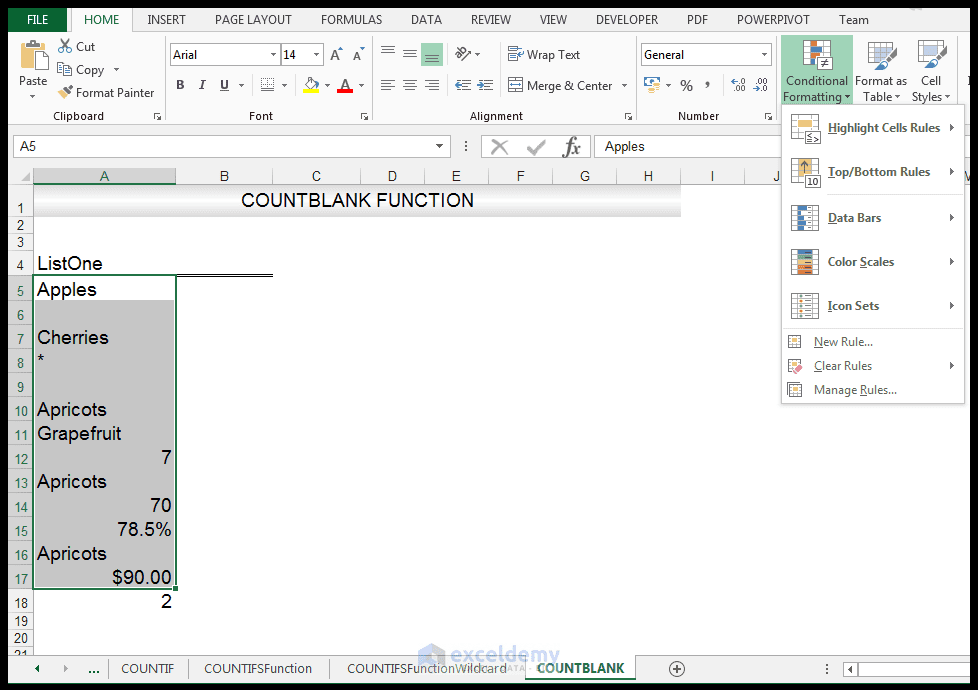3) Go to Highlight Cells Rules>More rules…….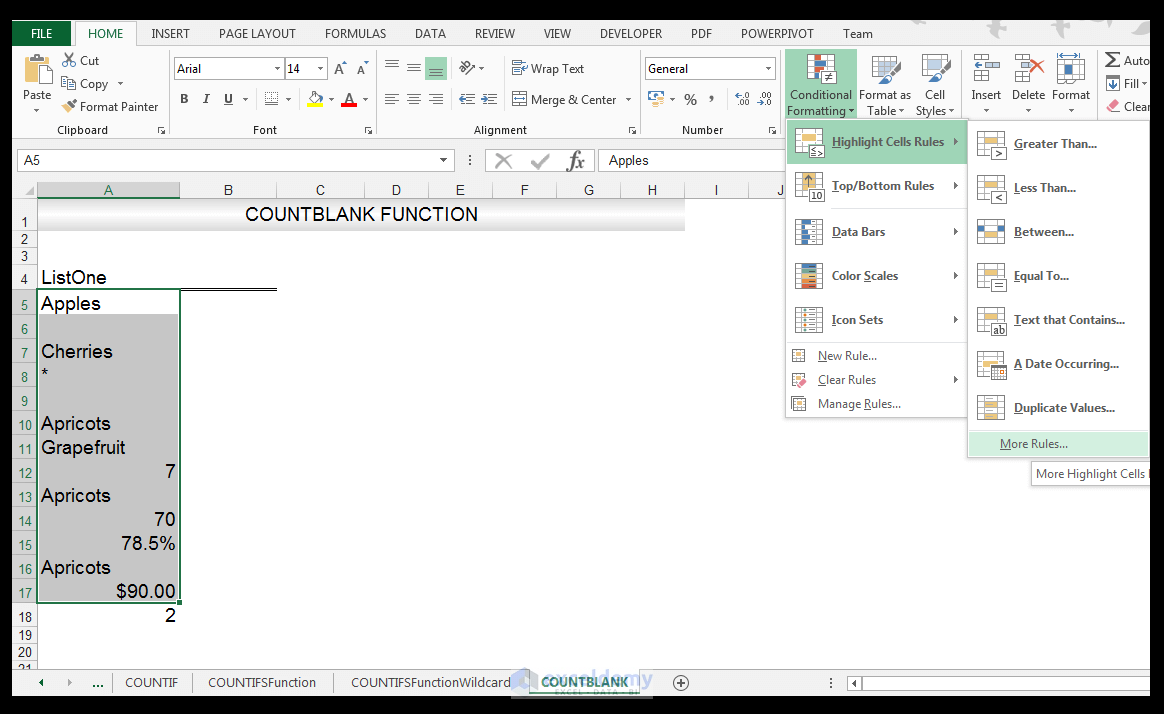4) In the New Formatting Rule dialog box, choose Format only cells that contain and then Format only cells with Blanks and choose a format, in this case, a blue fill. Click Ok.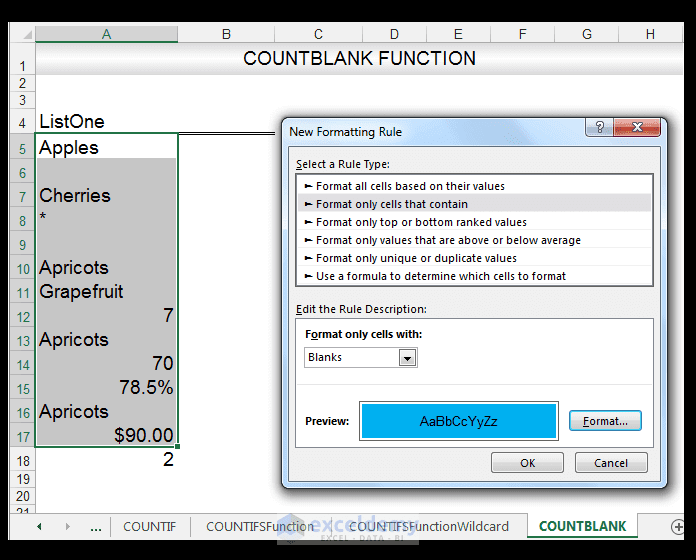5) All the blank cells, in the range, selected now have a blue fill.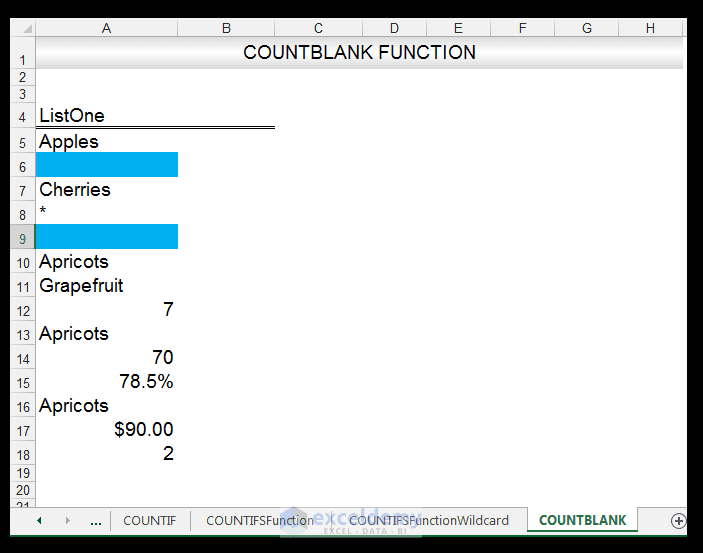And there you have it.

## Conclusion

It is often necessary to count different types of values or use diverse criteria when counting. Excel provides five different counting functions that can be used on their own or in combination with other functions in order to create advanced formulas. While the Word options for adding, counting or summing values are less, Word does have a few functions that one can use to perform operations on Table data. Wildcards can also be used to extend functionality, especially when using the COUNTING Functions. The three wildcards *,? and ~ have to still be surrounded by quotation marks in formulas but when utilizing them in formulas, they allow one to encompass a greater degree of flexibility or counting options in this case.

Please feel free to comment and tell us how you utilize counting functions, in your spreadsheets or Word documents.